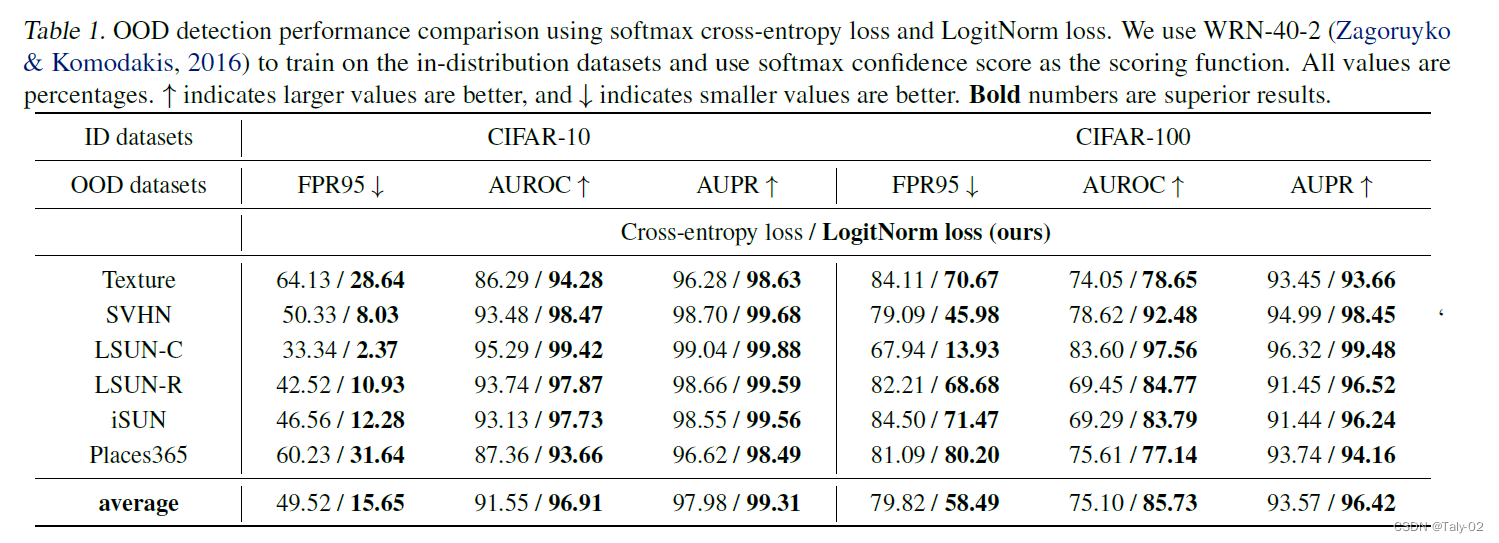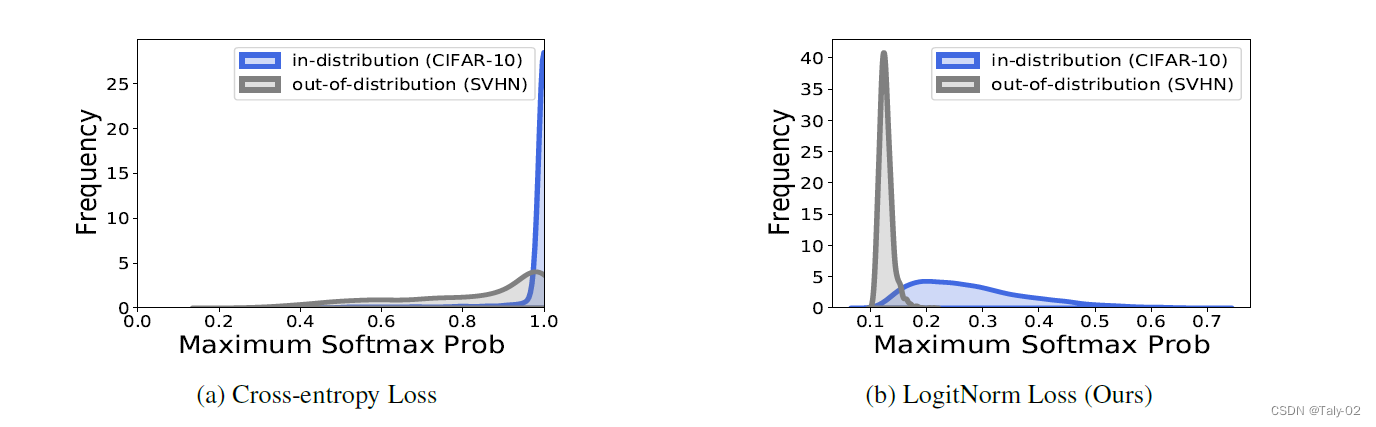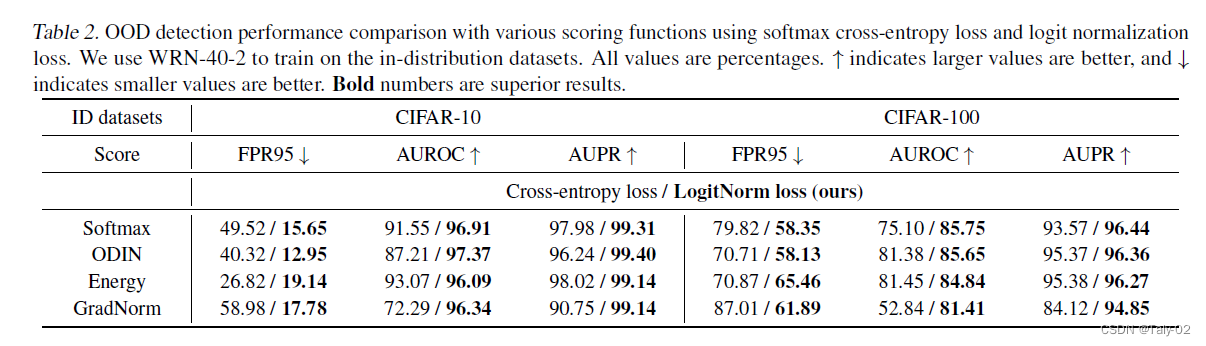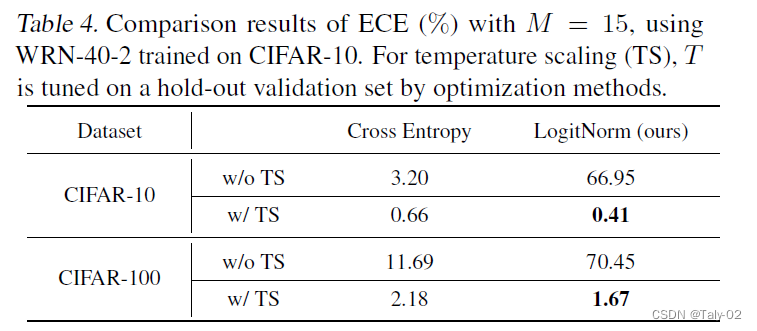# Mitigating Neural Network Overconfidence with Logit Normalization ICML 2022¶

### 2. 介绍¶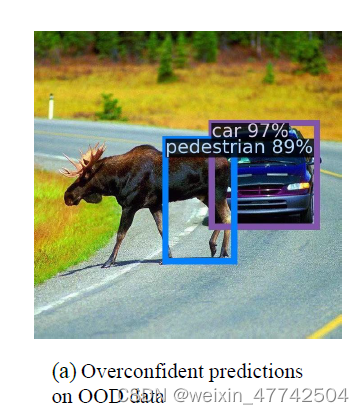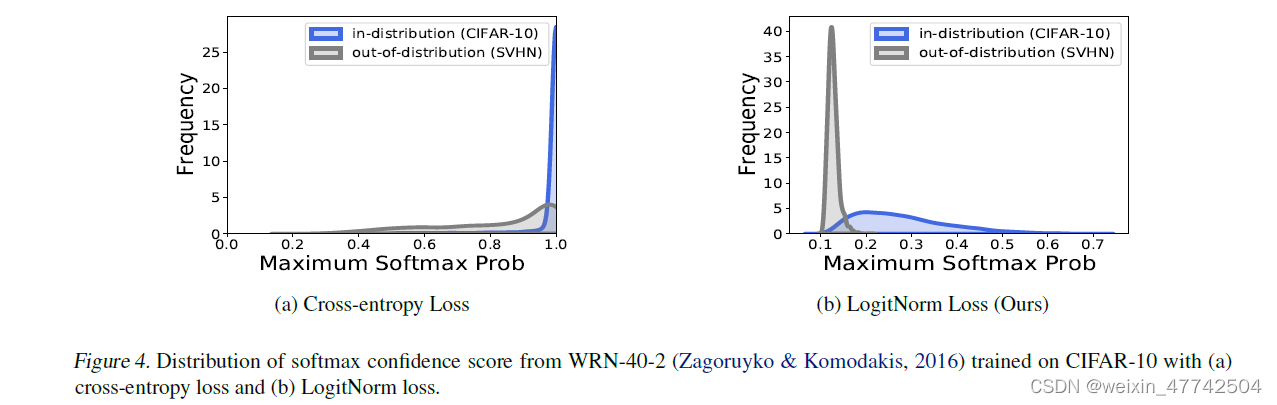### 3. 方法¶

f=||f|| \cdot \hat{f}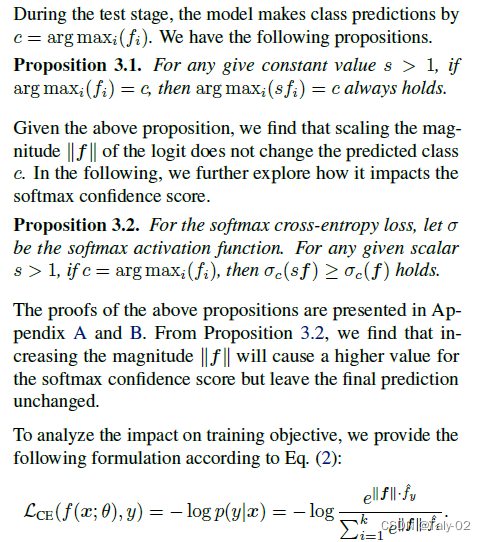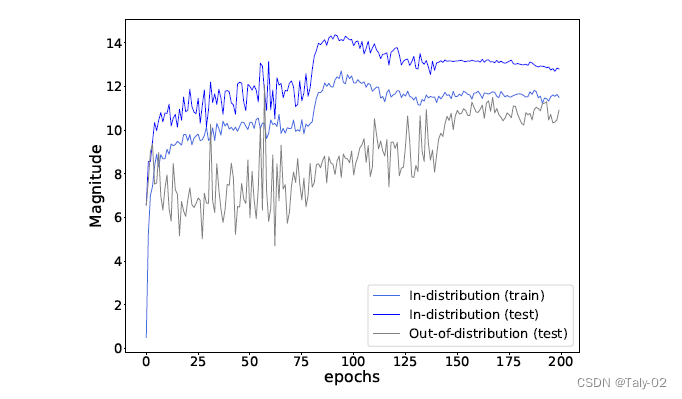\mathcal{L}_{\text {LogitNorm }}(f(x ; \theta), y)=-\log \frac{e^{f_{y} /(\tau\|\boldsymbol{f}\|)}}{\sum_{i=1}^{k} e^{f_{i} /(\tau\|\boldsymbol{f}\|)}}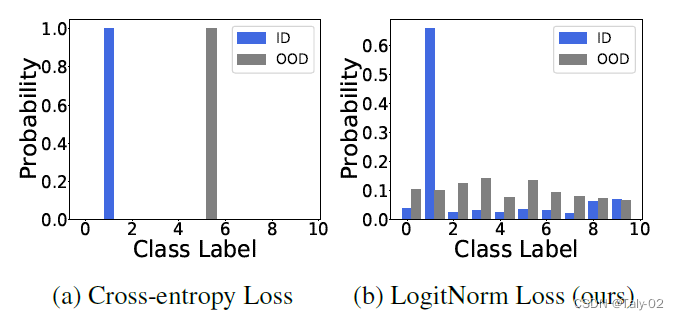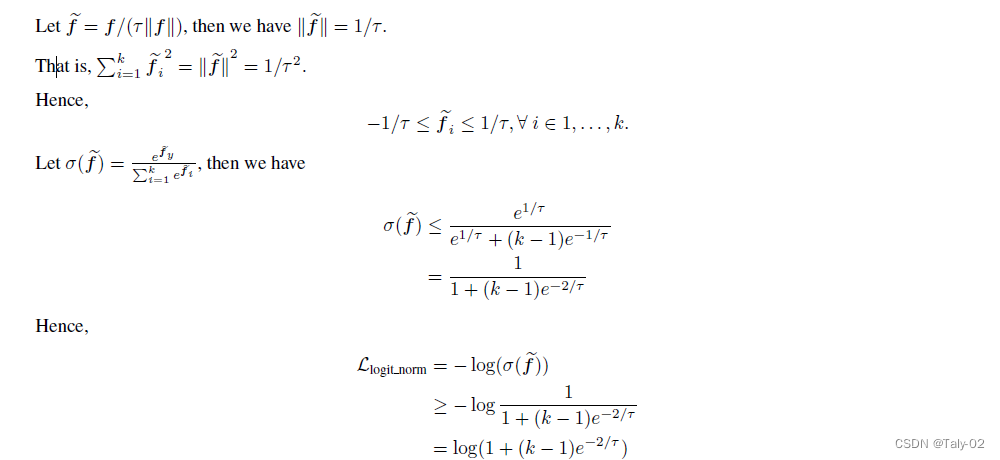可以发现，温度系数越大，损失函数下界也随之升高，所以说温度影响优化极限。

### 4. 实验¶